It is very easy to draw a circle if it is said to draw it by a compass or a protector but what if you need to draw it free hand? Can you draw a perfect round circle without going away from exact track? If no then let us see how to draw a circle freehand and let us learn everything about drawing circles freehand.

## How to Draw a Circle Freehand

### Understanding the Principle of Circle

Before drawing a circle or before going into the topic of how to draw a circle freehand let us first understand what the basic concept of circle is. A circle is round plain 2D figure which is constructed by numbers and numbers of points placed next to each other but equidistance from a single point called centre. For example consider the image below; here all the points on circumference are equidistance from centre point C.

Distance from C to all the points A, B, A’ and B’ are equal. This distance is called radius of a Circle and twice of this radius is called diameter. That’s all we should know before drawing circles.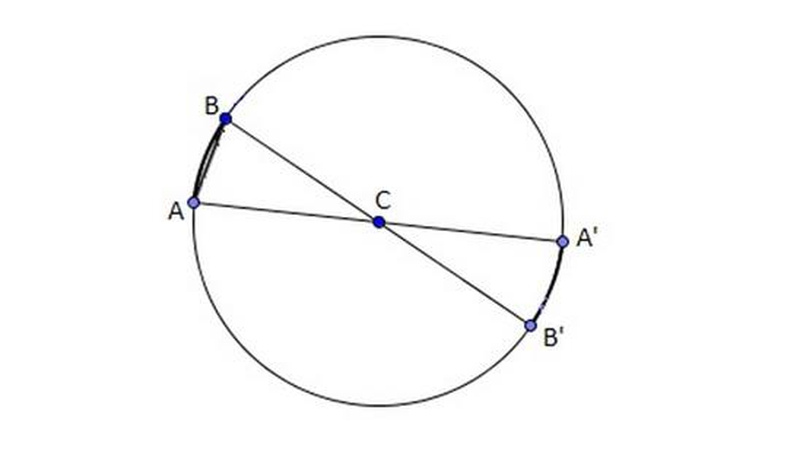### A Perfect Circle with Help of Your Wrist

without help of any substance or object. What we need is a sharpen pencil with book, copy or any paper where circle is to be drawn. First step is to clear all things from table and put wrist on paper such that it becomes center of the circle then put the nib point of pencil on paper without disturbing place of the wrist. Then just rotate the paper in anti-clockwise direction.

You will see a perfect circle on the paper. If you are lefty then rotate the paper in clockwise direction. Understanding this simple concept now you are must not wonder about how to draw a circle freehand.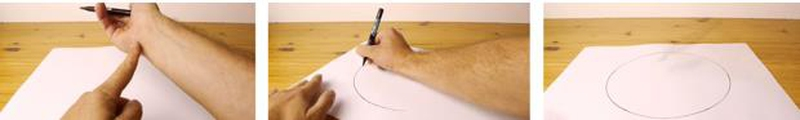### A Smaller Perfect Circle Using Your Fingers

Do you think that the circle you draw is much bigger than you actually want? Do not worry, now what you have to do is just make a smaller gap between the pencil and the point where you are pressing the paper. This can be completed with the help of your finger as shown in the image below. Press paper with the help of the backside of your folded little finger or middle finger and draw circle.### Draw a Circle in Gimp

If you are looking for instructions to learn how to draw a circle in gimp then you are at right place. There are only three steps to gimp draw circle. First, select a new layer in your image so that it allows you to draw circle. Second, select tool of Ellipse from the toolbox of gimp. Final step, draw ellipse or circle anywhere in the image. If you are still find it difficult then try to concentrate on movement of mouse.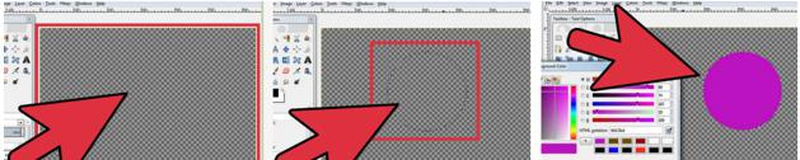### A Circle Freehand Without Using Any Support

It is very easy for anyone to draw a circle freehand if he/she knows the trick to draw it but what if you need to draw circle without any support of your wrist or fingers such as you do not find thing to be movable so, how could you rotate it?. It is not too tough to draw circles without any trick what one should do is first try to draw a small circle, take your time but try to draw accurate perfect circle.

After making a small one, draw a bigger circle with same centre point. Try practicing it but if you find hard to draw circle clockwise then try drawing anti-clockwise. Don’t be surprised if you find it the easiest as well as toughest method among all methods of how to draw a circle.### Drawing Circle on A Board

Are you a teacher and looking for a help to draw a perfect circle on board? Let us learn how to draw a circle on board. There is a very easy method to draw a perfect circle on board. Take a marker and stand against the board, use your elbow as centre of the circle and trace it by moving your arm 360 degrees clockwise or anticlockwise. If you want smaller circle than use your wrist as centre of the circle but this time you do not have to touch the board by wrist just presume it as a centre and draw the circle.

## Drawing Circle with Different Objects

If you still find hard to draw a circle freehand then you may use some of the following mathematical means of drawing circle. These objects are generally used if one wants a precise circle with accurate circumference such as gimp draw circle. After learning to draw circles freehand you may teach others about how to draw a circle freehand.

### Learn to Draw a Circle by Protector

It is normally used to measure angles between two lines cutting each other from some point but the shape of protector is exactly as semicircle. So if you want to draw circles then you may use it by drawing first half of circle then another half of the circle. One drawback of tracing circle by it is that you can’t alter size of it to trace the circle.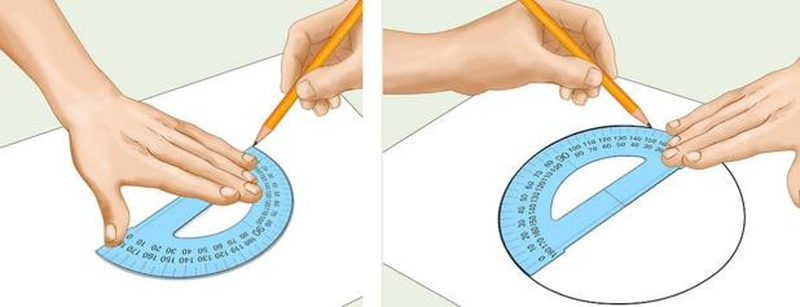### By Compass

It is mostly used for drawing circles so let us learn how to draw a circle with the help of it. It is meant to trace a circle but a mathematical person can also use it to trace different angles for technical drawing. With the help of this you can easily draw any size of circle, what you need to do is attach a pencil on one end and put the other end as centre of the circle and draw circle according you your desired size by altering the size of the radius of circle. If you still not able to learn how to draw a circle freehand then you may opt this option for drawing perfect circles in no time.### Ruler

It is a very time consuming method for drawing circles with the help of ruler. In this method you first need to locate centre of the circle you are drawing. Then take a ruler and put dots equidistance from centre then trace a curve joining all the points. It works on the basic definition of Circle. If you do not want to waste time in this time consuming method then it is better to learn how to draw a circle freehand.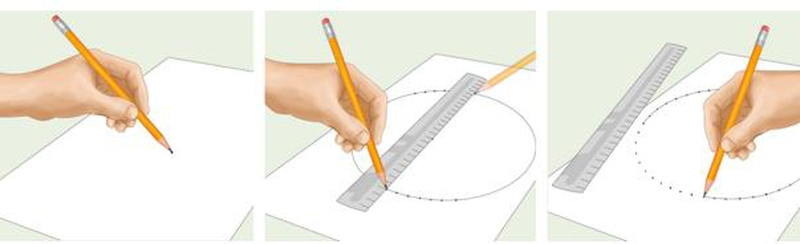### String

It is the oldest method used to trace circles and the circles drawn by this method are as accurate as gimp draw circle. Have you seen the runner’s path in Olympics or in any running sports event? That Curve of the running track is traced with the help of this method. You must be excited to learn how to draw a circle by this method as it is the most ancient method used to draw a circle.

In this method a string is connected to both centre point and marker. Then by keeping centre point still marker is moved around it with the distance same as string. The string holds it not to go away and perfect circle gets traced.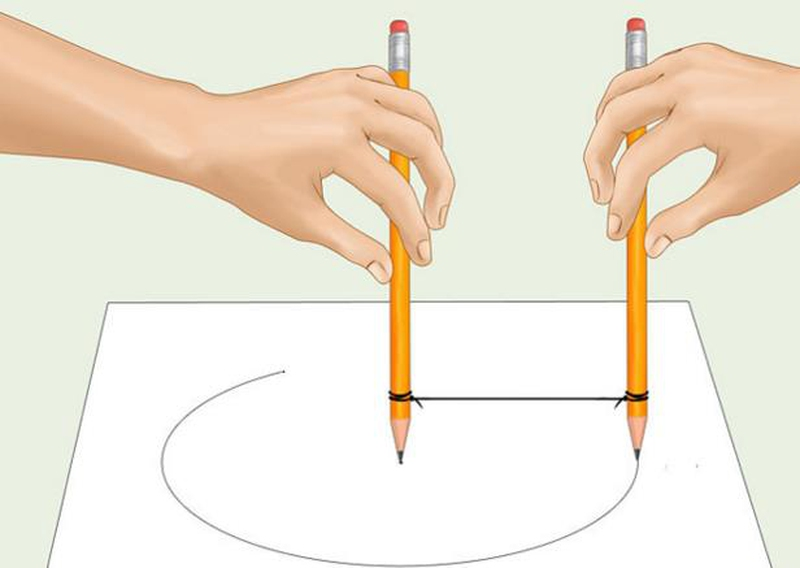## Final Word

### Conclusion

There are many objects available so that we do not find it important to learn how to draw a circle freehand but many times we fail to find materials for drawing circles. At such timings it becomes necessary for us to trace a circle on our own. Drawing a circle freehand might be a little time consuming but makes you perfect in drawing because if you learn how to draw circle freehand then you would know how to draw curves in drawing.

This is the reason kids are taught to draw lines and circles first. So what you are waiting for try it for yourself and you’ll definitely find it interesting and useful.